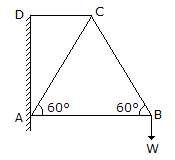Last updated: August 14, 2020

1. The angle of inclination of the plane at which the body begins to move down the plane, is called
2. The law of the machine is(where P = Effort applied to lift the load, m = A constant which is equal to the slope of the line, W = Load lifted, and C = Another constant which represents the machine friction.)
3. If P is the force acting on the body, m is the mass of the body and a is the acceleration of the body, then according to Newton's second law of motion,
4. The total motion possessed by a body, is called
5. The forces, which do not meet at one point, but their lines of action lie on the same plane, are known as coplaner non-concurrent forces.
6. The forces, which meet at one point and their lines of action also lie on the same plane, are known as
7. The matter contained in a body, is called
8. The amount by which the outer edge of the rail is raised, is known as super-elevation.
9. The potential energy stored by a spring in compression, is called strain energy.
10. If the resultant of a number of forces acting on a body is zero, then the body will be in equilibrium.
11. The S.I. unit of energy is
12. The centre of percussion is below the centre of gravity of the body and is at a distance equal to
13. The moment of a force
14. The friction experienced by a body, when at rest, is known as
15. If a number of coplaner forces acting at a point be in equilibrium, the sum of clockwise moments must be __________ the sum of anticlockwise moments, about any point.
16. The frequency of oscillation of a compound pendulum is(where kG = Radius of gyration about the centroidal axis, and h = Distance between the point of suspension and C.G. of the body.)
17. The angle which the normal reaction makes with the resultant reaction is called angle of friction.
18. In a single threaded worm and worm wheel, the number of teeth on the worm is 50. The diameter of the effort wheel is 100 mm and that of load drum is 50 mm. The velocity ratio is
19. The moment of inertia of a solid sphere of mass m and radius r is
20. In a framed structure, as shown in the below figure, the force in the member AB is __________ the force in member AC.Engineering Mechanics MCQ 11
You got {{userScore}} out of {{maxScore}} correct
{{title}}
{{image}}
{{content}}